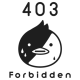tzippy - 2 years ago 126
Java Question

# Java: Check if two double values match on specific no of decimal places

Comparing those two values shall result in a "true":

``````53.9173333333333  53.9173
``````kennytm

If you want `a = 1.00001` and `b = 0.99999` be identified as equal:

``````return Math.abs(a - b) < 1e-4;
``````

Otherwise, if you want `a = 1.00010` and `b = 1.00019` be identified as equal, and both `a` and `b` are positive and not huge:

``````return Math.floor(a * 10000) == Math.floor(b * 10000);
// compare by == is fine here because both sides are integral values.
// double can represent integral values below 2**53 exactly.
``````

Otherwise, use the `truncate` method as shown in http://stackoverflow.com/questions/1976809/are-there-any-functions-for-truncating-a-double-in-java:

``````BigDecimal aa = new BigDecimal(a);
BigDecimal bb = new BigDecimal(b);
aa = aa.setScale(4, BigDecimal.ROUND_DOWN);
bb = bb.setScale(4, BigDecimal.ROUND_DOWN);
return aa.equals(bb);
``````
Recommended from our users: Dynamic Network Monitoring from WhatsUp Gold from IPSwitch. Free Download